• } 时间复杂度分析 使用master公式 T(N)=2T(N/2)+O(N) a=2 b=2 d=1 符合log b a=d 所以归并排序的时间复杂度为O(N*logN) 02归并排序拓展 小和问题与逆序对问题 小和问题 在一个数组中，每一个数左边比当前数小的数...

01归并排序

归并排序就是先将一个数组的左侧与右侧都有序，然后用两个指针分别比较两个数组元素的大小，将比较结果复制到辅助数组中。
整体采用递归方法，确定递归基是需要排序的序列只有一个数的时候。其余情况，则需要不断向下继续递归。

void mergesort(int l,int r,int arr[])
{
if(l == r)
return;
int m = l+(r-l)>>1;
mergesort(l,m,arr);
mergesort(m+1,r,arr);
merge(l,m,r,arr);
}

如果l=r的时候，说明需要排序的序列只有一个数，必然是有序的，所以直接返回。
接下来看merge方法，将两个有序序列合并成为一个有序序列的过程。

void merge(int l,int m,int r,int arr[])
{
int help[ARR_MAX];
int i = 0;
int p1 = l;
int p2 = m + 1;
while(p1<=m &&p2<=r)
{
help[i++] = (arr[p1]>arr[p2]? arr[p2++]:arr[p1++]);
}
while(p1<=m)
{
help[i++] = arr[p1++];
}
while(p2<=r)
{
help[i++] = arr[p2++] ;
}
for(i = 0; i < r-l+1; i++){
arr[l+i] = help[i];
}
}

1. 开辟一个辅助数组，大小与原数组相同，该数组的元素用一个指针位置i来表示。
用p1代表左侧序列的下标位置，初始值就是左边界l，最大值是m，
p2代表右侧序列的下标位置，初始值就是m+1，最大值是r。
2. 若两个序列的指针都没有超过最大值
若左侧序列的对应元素小于等于右侧序列的对应元素，就将左侧序列的对应元素复制到辅助数组中。若左侧对应数组大于右侧序列的对应元素，则将右侧序列的对应元素复制到辅助数组中。这一过程中，复制元素的序列和辅助数组，对应的指针位置都需要后移。
3. 若有一个序列的指针越界，则需将另一个序列中剩余的元素都依次复制到辅助数组中。
4. 最终将辅助数组中的元素，复制到原数组中。

完整测试代码

#include <iostream>
#include<time.h>
#define B_MAX 7
using namespace std;

void merge(int l, int m, int r, int* b)
{
int help[B_MAX];
int i = 0;
int p1 = l;
int p2 = m + 1;
while (p1 <= m && p2 <= r)
{
help[i++] = (b[p1] > b[p2] ? b[p2++] : b[p1++]);
}
while (p1 <= m)
{
help[i++] = b[p1++];
}
while (p2 <= r)
{
help[i++] = b[p2++];
}
for (i = 0; i < r - l + 1; i++) {
b[l + i] = help[i];
}
}

void mergesort(int l, int r, int* b)
{
if (l == r)
return;
int m = (r + l) / 2;
mergesort(l, m, b);
mergesort(m + 1, r, b);
merge(l, m, r, b);
}

int main()
{
srand((unsigned)time(NULL));
int b[B_MAX];
for (int i = 0; i < B_MAX; i++)
{
b[i] = rand() % 10;
cout << b[i] << ' ';
}
cout << endl;
mergesort(0, B_MAX - 1, b);
for (int i = 0; i < B_MAX; i++)
{
cout << b[i] << ' ';
}
system("pause");
return 0;
}

时间复杂度分析
使用master公式
T(N)=2T(N/2)+O(N)
a=2 b=2 d=1
符合log b a=d 所以归并排序的时间复杂度为O(N*logN)

02归并排序拓展

小和问题与逆序对问题

小和问题

在一个数组中，每一个数左边比当前数小的数累加起来，叫做这个数组的小和。求一个数组的小和。
例子 1,3,4,2,5
1左边比1小的数，没有；
3左边比3小的数，1;
4左边比4小的数，1,3;
2左边比2小的数，1;
5左边比5小的数，1,3,4,2;
所以小和是这些数累加起来和为16
分析：求小和就是遍历当前数组，选择一个数，让这个数与左边的数依次比较，若左边的数小于当前数，则将该数累加到小和结果中。换种情况来考虑问题，求小和就是看当前数的右侧有几个数比当前数大，那么就加几倍的当前数。换个角度分析之后，时间复杂度还是一样的，我们使用归并排序的思想，使得在两组序列merge的时候，计算当前的小和。因为这两个系列都是有序的，所以不需要全部遍历，只需要计算右侧序列的下标即可。

while (p1 <= m && p2 <= r) {//都不越界的时候
res += arr[p1] < arr[p2] ? (r - p2 + 1) * arr[p1] : 0;
//只有左组比右组小，才产生小和数量增加的行为，当前右组有多少个数比p1大
help[i++] = arr[p1] < arr[p2] ? arr[p1++] : arr[p2++];
}

在排序的同时记录小和结果，当右侧序列数大于当前左侧序列数的时候，小和增加(r - p2 + 1)倍的当前左侧数。当左组与右组的数相等时，需要先向下拷贝右组的数，因为不这样，不知道右组有几个数比当前数大。
完整代码

#include<iostream>
#define ARR_Length 5
using namespace std;
int merge(int l, int m, int r, int *b)
{
int help[ARR_Length];
int p1 = l;
int p2 = m + 1;
int i = 0;
int result = 0;
while (p1 <= m && p2 <= r)
{
result += b[p1] < b[p2] ? ((r - p2 + 1) * b[p1]) : 0;
help[i++] = b[p1] > b[p2] ? b[p2++] : b[p1++];
}
while (p1 <= m)
{
help[i++] = b[p1++];
}
while (p2 <= r)
{
help[i++] = b[p2++];
}
for (i = 0; i < r - l + 1; i++)
{
b[i + l] = help[i];
}
return result;
}

int mergesort(int l, int r, int *b)
{
if (l == r)
return 0;
int m = l + ((r - l) >> 1);

return mergesort(l, m, b) //左侧排好并取小和
+ mergesort(m + 1, r, b) //右侧排好并取小和
+ merge(l, m, r, b);//merge时产生小和
}

int main()
{
int b[ARR_Length] = { 1,3,4,2,5 };
int a = 0;
a = mergesort(0, ARR_Length - 1, b);
cout << a;
return 0;
}

逆序对

在一个数组中，左边的数如果比右边的数大，则这两个数构成一个逆序对，请打印所有的逆序。
分析：找逆序的过程就是在归并的过程中，如果左边的数大于右边的数，那么计算左侧序列的下标，记录逆序数，然后将逆序数都打印出来
完整代码

#include<iostream>

#define B_Length 5
using namespace std;
int merge(int l, int m, int r, int b[])
{
int help[B_Length];
int p1 = l;
int p2 = m + 1;
int i = 0;
int result = 0;
while (p1 <= m && p2 <= r)
{
result += b[p1] > b[p2] ? (m - p1 + 1) : 0;
if (b[p1] > b[p2])
for (int i = p1; i <= m; i++) {
cout << b[i] << b[p2] << endl;
}
help[i++] = b[p1] > b[p2] ? b[p2++] : b[p1++];
}
while (p1 <= m)
{
help[i++] = b[p1++];
}
while (p2 <= r)
{
help[i++] = b[p2++];
}
for (i = 0; i < r - l + 1; i++)
{
b[i + l] = help[i];
}
return result;
}

int mergesort(int l, int r, int b[])
{
if (l == r)
return 0;
int m = l + ((r - l) >> 1);

return mergesort(l, m, b) + mergesort(m + 1, r, b) + merge(l, m, r, b);
}

int main()
{
int b[B_Length] = { 1,3,4 ,2,5 };
int a = 0;
a = mergesort(0, B_Length - 1, b);
cout << a;
system("pause");
return 0;
}

03堆

1. 堆结构就是用数组实现的完全二叉树结构。
2. 完全二叉树中如果每棵树的最大值在顶部就是大根堆
3. 完全二叉树中如果每棵树的最小值在顶部就是小根堆
4. 堆结构的heapinsert和heapify操作
5. 堆结构的增大和减小
6. 优先级队列结构就是堆结构

什么是完全二叉树？二叉树是的，若是不满也是从左到右依次变满
数组从0出发的连续一段可以对应成完全二叉树（数组元素按二叉树行依次添加）
堆结构节点与数组对应位置关系
左孩子节点为2i+1，右孩子节点为2i+2 父节点为（i-1）/2

heapInsert：新节点插入进来，并向上调整形成大根堆的过程

分析：某个数现在处在index位置，往上继续移动，将当前数与父位置的数进行比较，若当前数大于父位置的数，则两个数交换位置，index更新到刚才父节点的位置。

//某个数现在处在index位置，往上继续移动
void heapinsert(int arr[],int index)
{
while(arr[index] > arr[(index-1)/2])//若当前的数大于父位置的数
{
swap(arr[index],arr[(index-1)/2]);
index = (index-1)/2;//换完后来到父位置，继续在循环中判断 直到不比父节点大 或到达根节点
}
}

使用for循环遍历数组，调用heapinsert

for(int i = 0;i < length;i++ )
{
heapinsert(arr,i);//用for循环传入要处理的index
}

时间复杂度分析
插入一个数需要比较数的高度次，也就是O（logN），N个数需要比较log1+log2+…+log（N-1）=O(N)

heapify 假设数组中某个值变小，重新将数组调整为大根堆的过程

分析：找到这个数的左右孩子的最大值，将左右孩子的最大值与这个数进行比较，
若孩子节点大于父节点，交换位置。
停止条件：左右孩子的最大值小于等于父节点或者 没有左右节点

//某个数在index位置，能否往下移动
void heapify(int arr[],int index,int heapsize)
{
int left = index * 2 + 1; //左孩子的下标
while(left < heapsize)
{	//两个孩子中，谁的值大，把下标给large
int largest = left + 1 < heapsize && arr[left]<arr[left + 1] ?
left + 1:left;
//父亲和较大孩子之间，谁的值大，就把下标给largest
largest = arr[largest] > arr[index] ? largest : index;
if(largest == index)//父节点就是三个节点中的最大值
break;
swap(arr[largest],arr[index]);
index = largest;//向下移动
left =  index * 2 + 1;
}
}

04堆排序

1. 先让整个数组都变成大根堆结构，建立堆的过程
1.1 从上到下的方法，时间复杂度O(N*logN)（0-0位置是大根堆，依次插入数组元素进行heapInsert）
1.2 从下到上的方法，时间复杂度为O(N)（先让最底层的节点heapify，再让上一层的，不断依次向上heapify）
2. 把堆的最大值与堆末尾的值交换，然后减少堆的大小后，再去重新调整堆为大根堆，一直周而复始，时间复杂度为O(N*logN)
3. 堆的大小减小为0的时候，排序完成。

排序代码

void heapsort(int arr[],int heapsize)
{
while(heapsize > 0)
{
heapify(arr,0,heapsize);//O(logN) 0位置数往下heapify
swap(arr,arr[--heapsize]);//O(1) 0位置与当前末位置交换
}
}

堆排序完整代码

#include<iostream>
#include<time.h>
#define length 10
using namespace std;

void swap(int& a, int& b)
{
int temp = a;
a = b;
b = temp;
}

void heapify(int arr[], int index, int heapsize)
{
int left = 2 * index + 1;
while (left < heapsize)
{
int largest = left + 1 < heapsize && arr[left + 1] > arr[left] ?
left + 1 : left;
largest = arr[index] < arr[largest] ? largest : index;
if (index == largest)
break;
swap(arr[index], arr[largest]);
index = largest;
left = 2 * index + 1;
}

}

void heapsort(int arr[], int heapsize)
{
while (heapsize > 0)
{
heapify(arr, 0, heapsize);//O(logN) 0位置数往下heapify
swap(arr, arr[--heapsize]);//O(1) 0位置与当前末位置交换
}
}

void heapinsert(int arr[], int index)
{
while (arr[index] > arr[(index - 1) / 2])
{
swap(arr[index], arr[(index - 1) / 2]);
index = (index - 1) / 2;
}
}

int main()
{
srand((unsigned)time(NULL));

int arr[length];
int heapsize = length;
cout << "arr = ";
for (int i = 0; i < heapsize; i++)
{
arr[i] = rand() % 10;
cout << arr[i] << " ";
heapinsert(arr, i);
}
cout << endl << "arr1 = ";

heapsort(arr, heapsize);

for (int i = 0; i < length; i++)
{
cout << arr[i] << " ";
}
cout << endl;

//system("pause");
return 0;
}

05堆排序扩展题目

已知一个几乎有序的数组，几乎有序是指如果数组排好顺序的话，每个元素移动的距离可以不超过k，并且k相对于数组来说比较小。请选择一个合适的排序算法针对这个数据进行排序。
分析：将0-k位置的数置为小根堆，0位置必然是小根堆的最小值，将0位置弹出，将k+1位置的数插入，每次添加一个数就弹出一个数，没有数需要插入时，依次弹出小根堆中的数

排序java代码

public void sortedArrDistanceLessK(int[] arr, int k) {
PriorityQueue<Integer> heap = new PriorityQueue<>();//默认为小根堆
int index = 0;
for (; index <= Math.min(arr.length, k); index++) {//把前k+1个数放到小根堆
}
int i = 0;
for (; index < arr.length; i++, index++) {//每次添加一个数，弹出一个数
arr[i] = heap.poll();
}
while (!heap.isEmpty()) {//没有数需要添加后，依次弹出小根堆中的数
arr[i++] = heap.poll();
}
}

06荷兰国旗问题

问题一

给定一个数组arr，和一个数num，请把小于等于num的数放在数组的左边，大于num的数放在数组的右边。要求额外空间复杂度O(1)，时间复杂度O(N)

分析：开辟一个小于等于num的区域，然后将这个区域的初始位置置于数组边界左侧，通过与数组元素的比较的过程，小于等于区域不断推着大于区域向右移动，直到将数组元素遍历完。
具体的比较规则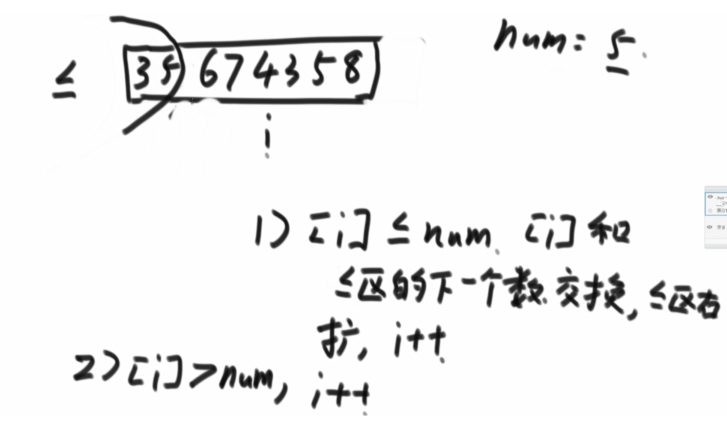代码

#include<iostream>
#include<time.h>
#define arr_length 10
using namespace std;

//交换函数
void swap(int &a,int &b)
{
int temp = a;
a = b;
b = temp;
}

int main()
{
int arr[arr_length];
int cur = 0;
int num = 6;
int x = -1;

//生成随机长度为arr_length的数组，并输出
srand((unsigned)time(NULL));
cout<<"arr = ";
for(int i = 0;i < arr_length;i++)
{
arr[i] = rand()%10;
cout<<arr[i]<<" ";
}
cout<<endl;

//核心代码
while(cur < arr_length)
{
if(arr[cur] <= num)
{
swap(arr[cur++],arr[++x]);
}
else
{
cur++;
}
}

//输出交换后的arr1，arr2
cout<<"arr1 = ";
for(int i = 0;i<=x;i++)
{
cout<<arr[i]<<" ";
}
cout<<endl;
cout<<"arr2 = ";
for(int i = x+1;i<arr_length;i++)
{
cout<<arr[i]<<" ";
}
cout<<endl;
system("pause");
return 0;
}

问题二

给定一个数组arr，和一个数num，请将小于num的数放在数组的左边，等于num的数放在数组的中间，大于num的数放在数组的右边。要求额外空间复杂度O(1)，时间复杂度O(N)

分析：再看问题二，两个问题的区别就是问题二中需要将等于num的数放在中间，那么我们可以在问题一的基础上再开辟一个大于num的区域，位于数组的右侧。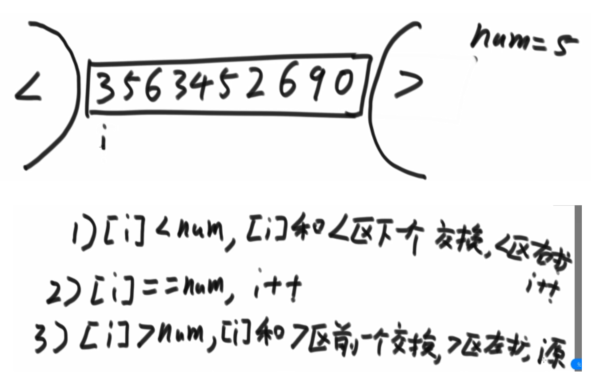小于区域推着等于区域奔向大于区域，大于区域往左压缩待定位置
代码

#include<iostream>
#include<time.h>
#define arr_length 5
using namespace std;

//交换函数
void swap(int &a,int &b)
{
int temp = a;
a = b;
b = temp;
}

int main()
{
int arr[arr_length];
int cur = 0;
int num = 6;
int x = -1;
int y = arr_length;

//生成随机长度为arr_length的数组，并输出
srand((unsigned)time(NULL));
cout<<"arr = ";
for(int i = 0;i < arr_length;i++)
{
arr[i] = rand()%10;
cout<<arr[i]<<" ";
}
cout<<endl;

//核心代码
while(cur < y)
{
if(arr[cur] < num)
{
swap(arr[cur++],arr[++x]);
}
else if(arr[cur] == num)
{
cur++;
}
else
{
swap(arr[cur],arr[--y]);
}
}

//输出交换后的arr1，arr2,arr3
cout<<"arr1 = ";
for(int i = 0;i<=x;i++)
{
cout<<arr[i]<<" ";
}
cout<<endl;
cout<<"arr2 = ";
for(int i = x+1;i<y;i++)
{
cout<<arr[i]<<" ";
}
cout<<endl;
cout<<"arr3 = ";
for(int i = y;i<arr_length;i++)
{
cout<<arr[i]<<" ";
}
cout<<endl;

system("pause");
return 0;
}

07不改进的快速排序

快排1.0

基于问题一，整个数组中，拿最后一个数当做num
按规则划分区域后，将大于区域的第一个数与当前数（最后一个数 ）交换位置
然后让左侧和右侧重复这个操作，每次递归都会有一个数排好位置
代码

#include<iostream>
#include<time.h>
#define length 10
using namespace std;

//交换函数
void swap(int &a,int &b)
{
int temp = a;
a = b;
b = temp;
}

int partition(int arr[],int l,int r)
{
int num = arr[r];	//把最后一个数设为num进行比较
int x = l-1;		//x为小于等于的区域，设为区域之前一个位置
int cur = l;		//cur是当前遍历的指针
while(cur < r+1)	//遍历所有位置
{
if(arr[cur]<=num)
{
swap(arr[cur++],arr[++x]);
}
else
cur++;
}

return x-1;
}

void quicksort(int arr[],int l,int r)
{
if(l<r)
{
int a = partition(arr,l,r);
quicksort(arr,l,a);
quicksort(arr,a+1,r);
}
}

int main()
{

//准备随机数组
int arr[length] ;
srand((unsigned)time(NULL));
cout<<"arr = ";
for(int i = 0;i < length;i++)
{
arr[i] = rand()%10;
cout<<arr[i]<<" ";
}
cout<<endl;

//意外情况直接返回(本题用不到)
if (arr == NULL || length < 2)
{
return 0 ;
}

//核心
quicksort(arr,0,length - 1);

//结果输出
cout<<"arr = ";
for(int i = 0;i<length;i++)
{
cout<<arr[i]<<" ";
}
cout<<endl;

system("pause");
return 0 ;
}

快排2.0

分析快排1.0的缺点：每次只能找出一个等于num的数进行排序，如果存在多个相同的num还需要继续划分，多做了很多无用功。基于问题二荷兰国旗，每次可以搞定一批等于num的位置，会使时间复杂度的常数项大大降低。
代码

#include<iostream>
#include<time.h>
#define length 20
using namespace std;

void swap(int &a,int &b)
{
int temp = a;
a = b;
b = temp;
}

int* partition(int arr[],int l,int r,int a[])
{
int x = l-1;
int y = r+1;
int cur = l;
int num = arr[r];
while(cur<y)
{
if(arr[cur]<num)
{
swap(arr[cur++],arr[++x]);
}
else if(arr[cur]>num)
{
swap(arr[cur],arr[--y]);
}
else
{
cur++;
}

}
a = x;
a = y;
return a;
}

void quicksort(int arr[],int l,int r)
{
int a = {0};
if(l<r)
{
int *p = partition(arr,l,r,a);
quicksort(arr,l,*p);
quicksort(arr,*(p+1),r);
}
}

int main()
{
//生成随机数组
int arr[length];
srand((unsigned)time(NULL));
cout<<"arr = ";
for(int i = 0;i<length;i++)
{
arr[i] = rand()%10;
cout<<arr[i]<<" ";
}
cout<<endl;

quicksort(arr,0,length - 1);

//打印结果
cout<<"arr1 = ";
for(int i = 0;i<length;i++)
{
cout<<arr[i]<<" ";
}
cout<<endl;

system("pause");
return 0;
}

08 随机快速排序

分析：荷兰国旗的快排2.0版本的缺点在于使用最后一个数作为num，那么此时的复杂度便于数据状况有关，若原数组的本来就是有序的，那么此时的复杂度便很高。如果从数组元素中随机选择一个数，就可以绕开原始数据状况，时间复杂度变为长期的期望值。
选择数据组随机一个数int num = arr[l+rand()%(r-l+1)];
代码

#include<iostream>
#include<time.h>
#define length 20

using namespace std;
void swap(int &a,int &b)
{
int temp = a;
a = b;
b = temp;
}
//这是一个处理arr[l..r]的函数
//返回一个长度为2的数组
int* partition(int arr[],int l,int r,int a[])
{
int x = l-1;//返回数组的左边界
int y = r+1;//返回数组的右边界
int cur = l;
int num = arr[l+rand()%(r-l+1)];//等概率随机选一个位置，与最右侧的数交换
while(cur<y)
{
if(arr[cur]<num)
{
swap(arr[cur++],arr[++x]);
}
else if(arr[cur]>num)
{
swap(arr[cur],arr[--y]);
}
else
{
cur++;
}

}
a = x;
a = y;
return a;
}

void quicksort(int arr[],int l,int r)
{
int a = {0};
if(l<r)
{
int *p = partition(arr,l,r,a);
quicksort(arr,l,*p);
quicksort(arr,*(p+1),r);
}
}

int main()
{
//生成随机数组
int arr[length];
srand((unsigned)time(NULL));
cout<<"arr = ";
for(int i = 0;i<length;i++)
{
arr[i] = rand()%100;
cout<<arr[i]<<" ";
}
cout<<endl;

quicksort(arr,0,length - 1);

//打印结果
cout<<"arr1 = ";
for(int i = 0;i<length;i++)
{
cout<<arr[i]<<" ";
}
cout<<endl;

system("pause");
return 0;
}

随机选择一个数，与最后一个数交换，然后开始划分区域。最好情况与最坏的情况都是概率事件。每种事件都是1/n，概率与事件复杂度相乘累加求得期望得时间复杂度是O(N*logN)额外空间复杂度为O(logN)

展开全文算法 排序算法
• 奇葩面试题，O(logn)的底数是多少？

千次阅读 多人点赞 2021-08-18 20:31:29
老三：O(logn)。 面试官：那这个复杂度的底数是多少？ 老三：时间复杂度O(logn)有底数？ 面试官：没有吗？ 尬住…… 面试官：那你再说一下快速排序的时间复杂度？底数是多少？ 老三露出智（尴）慧（尬）的微笑…… ...

大家好，我是老三，最近裸辞了，在面试。

前两天一个面试，只面了十分钟就结束了——

事情是这样的：

面试官：你能说说HashMap的数据结构吗？

老三：数组+链表+红黑树，阿巴阿巴……

面试官：那你说说红黑树的查找复杂度是多少？

老三：O(logn)。

面试官：那这个复杂度的底数是多少？老三：时间复杂度O(logn)有底数？

面试官：没有吗？

尬住……

面试官：那你再说一下快速排序的时间复杂度？底数是多少？

老三露出智（尴）慧（尬）的微笑……

面试官：好了，我没什么要问的了，这次面试到这结束吧。结束面试之后，老三意难平，赶紧查一下。

O(logn)是有底数的！

看一下时间复杂度的定义：

在进行算法分析时， 语句总的执行次数 T ( n ) 是关于问题规模 n 的 函 数 。 进 而 分 析 T ( n ) 随 n 的变化情况并确定 T ( n ) 的 数 量级。 算法的时间复杂度，也就是算法的时间量度， 记作： T ( n )= O(f(n))。它表示随问题规模 n 的增大， 算法执行时间的增长率和f ( n ) 的增长率相同， 称作算法的渐近时间复杂度， 简称为时间复杂度。 其中 f ( n ) 是问题规模 n 的某个函数。

有点抽象对不对，直接上例子，我们来意会一下。

int n=10;
int count=1;
while (count<n){
count=count*2;
//时间复杂度为O(1)的运算
System.out.println(count);
}

看一下，这个运算，每次 count 乘以 2 之后， 就距离n更近了一分。 也就是说：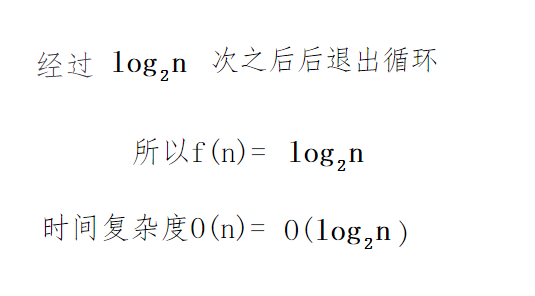破案了，O(logn)确实是有底数的。

这个底数是由什么决定的呢？

算法中log级别的时间复杂度都是由于使用了分治思想，这个底数直接由分治的复杂度决定。如果采用二分法,那么就会以2为底，，三分法就会以3为底数，其他类似。

O(logn)底数意义不大！

那问题来了，为什么我们平时不写底数呢？

总不能因为这个底数太难打吧……

我们注意到，时间复杂度的定义： T ( n )= O(f(n))。它表示随问题规模 n 的增大， 算法执行时间的增长率和f ( n ) 的增长率相同， 称作算法的渐近时间复杂度，简称时间复杂度。

假如说我们要比较两个函数f(n)和g(n)的增长快慢，用什么办法呢？

可以使用微积分里的极限：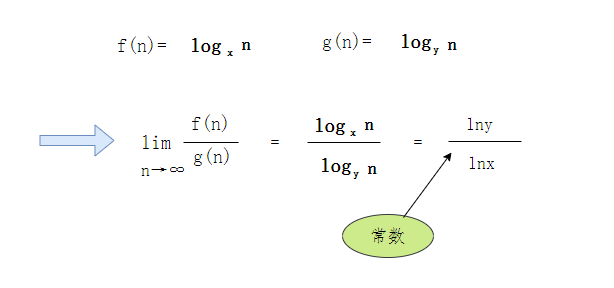老三高数忘完了哈哈，不会推导，总之最后的结果是一个常数。

也就是，假如n非常大的时候，任意底数的一个对数函数都只是相差一个常数倍而已。

所以无论底数是什么，log级别的渐进意义是一样的。也就是说该算法的时间复杂度的增长与处理数据多少的增长的关系是一样的。

总之：O（logn）已经可以表达所有底数的对数了

花了一个小时，无用的知识又增加了。

简单总结，就是O(logn)有底数，但是没有纠结的必要。

参考：

. 重学数据结构（序：概览）

展开全文算法
• 本文讲述时间复杂度为n*logn的排序算法：归并排序、快速排序、堆排序以及希尔排序的原理、Java实现以及变形应用。一、归并排序原理：把两个有序数列合并为一个有序数列。需递归实现。Java实现：1 public int[] ...

本文讲述时间复杂度为n*logn的排序算法：归并排序、快速排序、堆排序以及希尔排序的原理、Java实现以及变形应用。

一、归并排序

原理：把两个有序数列合并为一个有序数列。需递归实现。

Java实现：

1 public int[] mergeSort(int[] a, intn)2 {3 return doMergeSort(a, n, 0, n - 1);4 }5 public int[] doMergeSort(int[] a, int n, int start, intend)6 {7 int[] res = new int[n];8 if (n <= 1)9 {10 res =a[start];11 returnres;12 }13 //n>=2时，对其左右数组进行处理

14 int half = n / 2;15 int leftEnd = start + half - 1;16 int rightStart = leftEnd + 1;17 //递归调用本函数，获取有序的左数组以及右数组

18 int[] left =doMergeSort(a, half, start, leftEnd);19 int[] right = doMergeSort(a, n -half, rightStart, end);20 //将左右序列合并

21 int k = 0, i = 0, j = 0;22 while (i < half && j < n -half)23 {//由前往后比较两个序列，取较小值填充到res中，取值后该序列往后移动取下一个值比较

24 if (left[i] <=right[j])25 {26 res[k++] = left[i++];27 }28 else

29 {30 res[k++] = right[j++];31 }32 }33 //剩余的直接放入

34 while (i

二、快速排序

原理：每一次将一个数放在一个左边的数全部比它小，且右边的数全部比它大的位置，然后递归调用函数，将其左右序列排好。这边有一个比较好理解的做法：在数组的左边维护一个小于区间，在遍历的时候，发现比当前数小的数字的时候，将，扩增小于区间，并其放到小于区间内，结束后将当前数填充在小于区间后即可。

Java实现：

1 public int[] quickSort(int[] a, intn)2 {3 doQuickSort(a, n, 0, n - 1);4 returna;5 }6 public void doQuickSort(int[] a, int n, int start, intend)7 {8 if (n > 1)9 {10 int current =a[end];11 int minLen = 0;//小于区间的长度

12 int i =start;13 for (; i < end; i++)14 {15 if (a[i]

17 int temp = a[start +minLen];18 a[start + minLen] =a[i];19 a[i] =temp;20 minLen++;21 }22 }23 a[end] = a[start +minLen];24 a[start + minLen] =current;25 //当前位置已经确定，排左右序列

26 doQuickSort(a, minLen, start, start + minLen - 1);27 doQuickSort(a, n - minLen - 1, start + minLen + 1, end);28 }29 }

变形应用：三色排序练习题

有一个只由0，1，2三种元素构成的整数数组，请使用交换、原地排序而不是使用计数进行排序。给定一个只含0，1，2的整数数组A及它的大小，请返回排序后的数组。保证数组大小小于等于500。

测试样例：

[0,1,1,0,2,2],6

返回：[0,0,1,1,2,2]

解析：运用快排的原理。用数字1来处理，在数组左右各维护一个小于区间和大于区间。

三、堆排序

原理：维护一个大根堆(小根堆同理)，即维护一棵二叉树，该数子节点永远比父节点小。每次在大根堆中取出根，根为此时待排序列最大值，放在待排序列最后，然后调整大根堆，重复上诉过程即可。

Java实现：博主不太会插图，关于大小根堆的调整细节可自行百度。原理总结来说是从最后一个非叶子节点开始，往前调整。设当前调整的非叶子节点为n，选举n，n的左，n的右三个节点的最大值作为父节点。且每次调整了靠前的非叶子节点的值后，可能会破坏下面的数的大根堆规则，需要再次调整。嗯我觉得我并没有讲清楚，百度看看图就好。

public int[] heapSort(int[] a, intn)

{for (int len = n; len > 0; len--)

{//len为构建的堆的大小

for (int i = len / 2 - 1; i >= 0; i--)

{//从后往前遍历非叶子节点

while (2 * i + 1

{int j = 2 * i + 1;//左节点序号

if (j + 1 < len && a[j] < a[j + 1])

{

j++;

}if (a[i]

{int temp =a[j];

a[j]=a[i];

a[i]=temp;

i=j;

}else{break;

}

}

}int temp = a[len - 1];

a[len- 1] = a;

a =temp;

}returna;

}

变形应用：常规应用如在1000+个数中找出最大的10个数之类的。

已知一个几乎有序的数组，几乎有序是指，如果把数组排好顺序的话，每个元素移动的距离可以不超过k，并且k相对于数组来说比较小。请选择一个合适的排序算法针对这个数据进行排序。 给定一个int数组A，同时给定A的大小n和题意中的k，请返回排序后的数组。

测试样例： [2,1,4,3,6,5,8,7,10,9],10,2

返回：[1,2,3,4,5,6,7,8,9,10]

解析：维护一个大小为k的小根堆，每次调整完这个小根堆后，最小值就会出现在第一位，此时移除第一位，添加后一位数进来，继续调整这个小根堆即可。可以想象为一个从前往后移动的滑动窗口，滑动窗口中是一个小根堆。

四、希尔排序

原理：变形后的插入排序，每个数只与它前面固定步长的倍数的位置进行比对。如：步长step，当前数与它前面step，step*2，step*3....位置进行比较，插入到合适的位置。

Java实现：

public int[] shellSort(int[] a, intn)

{//步长选择

for (int k = 1, step = 10; step > 1; k++)

{

step= n / (2 *k);for (int i = step; i < n; i++)

{if (a[i] < a[i -step])

{int temp =a[i];

a[i]= a[i -step];

a[i- step] =temp;int pre = i -step;while (pre - step > 0)

{if (a[pre] < a[pre -step])

{

temp=a[pre];

a[pre]= a[pre -step];

a[pre- step] =temp;

pre-=step;

}else{break;

}

}

}

}

}returna;

}

展开全文• 什么是对数logN

千次阅读 2021-07-05 21:48:05
软件开发中通过合理的算法能减小计算的空间复杂度和时间复杂度，有的复杂度为logN，什么是logN？通过毕业后的努力工作基本都忘了。 对数 首先，logN被称为对数，是在幂函数的基础上衍生的。例如：3²=9，可以得到...

什么是对数

软件开发中通过合理的算法能减小计算的空间复杂度和时间复杂度，有的复杂度为logN，什么是logN？通过毕业后的努力工作基本都忘了。

对数

首先，logN被称为对数，是在幂函数的基础上衍生的。例如：3²=9，可以得到log以3为底的9的对数等于2，可以理解为3相乘等于9，这里是两个3相乘，所以结果就是2。

比较经典的二分查找法的时间复杂度就是O(logN)（以2为底），若是用迭代的方式时间复杂度是O（N），N是数据量的个数；我们可以假设若是个数有100个，每一次查询的时间用1毫秒，二分查找法最坏需要查询7毫秒，用迭代法最坏需要100毫秒。若是10亿次呢？二分查找法最坏只需要30毫秒，迭代法最坏需要277多个小时，这样一比较，高下立判，还是算法好。

平时开发中设计算法的时候大多是需要和业务强相关的场景，其他的例如排序和查找都已经有合适的工具类了，为了更好的应用指导算法原理是大有裨益的。

附一张《算法图解》中的截图。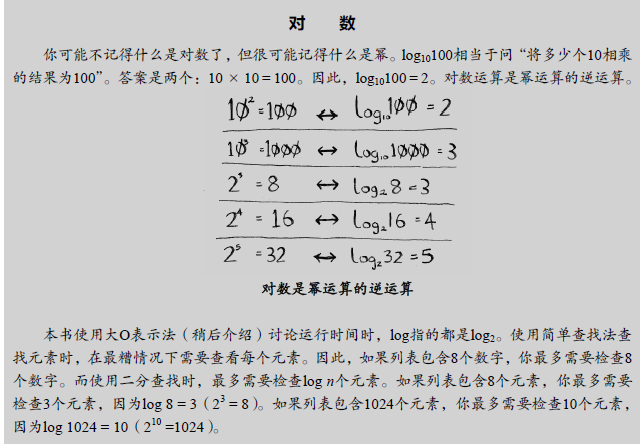电脑中计算机求对数

电脑中只有log（以10为底）和ln（以e为底）

根据换地公式计算：求以2为底8的对数，可以log8%log2=3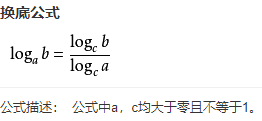展开全文• 关于算法的时间复杂度很多都用包含O(logN)这样的描述，但是却没有明确说logN的底数究竟是多少。 解答： 算法中log级别的时间复杂度都是由于使用了分治思想,这个底数直接由分治的复杂度决定。 如果采用二分法,那么就...算法 数据结构
• 斐波那契数列系列算法最优复杂度——时间复杂度优化到O(LogN) 对于菲薄那契系列问题的探讨很多，下面就以两道京东和阿里的面试真题来分析： 案例 一： 在迷迷糊糊的大草原上，小红捡到了n根木棍，第i根木棍的...
• 一.O(logn)代码小证明我们先来看下面一段代码:int cnt = 1;while (cnt < n){cnt *= 2;//时间复杂度为O(1)的程序步骤序列}由于cnt每次在乘以2之后都会更加逼近n，也就是说，在有x次后，cnt将会大于n从而跳出循环，...c语言的复杂度o1
• 快速排序：时间复杂度O(nlogn)，空间复杂度O(logn)，不稳定 归并排序：时间复杂度O(nlogn)，空间复杂度O(n)，稳定 堆排序：时间复杂度O(n*logn)，空间复杂度O(1)，不稳定 总结来说，归并排序的突出优势是稳定性，...
• 在描述算法复杂度时，经常用到O(1), O(n), O(logn), O(nlogn)来表示对应复杂度程度，可以用于表示时间复杂度，也用于表示空间复杂度。 类别 描述 O(1) 耗时与输入数据量大小无关 O(n) 数据量增大几倍，...算法 java 数据结构
• } O(n) 提示：递归的时间复杂度为其节点数，满二叉树层数为logn-1，节点数2^(m+1) - 1，所以到头来还是O(n) （4）递归真优化 int function3(int x, int n){ if (n == 0){ return 1; } t = function3(x, n/2) ...算法 递归法
• 起因是和一个帅哥（sb）讨论另一个问题，延伸到这个问题上面来的 怀着 对问题的严谨性我打开了尘封已久的pycharm ... x = ...python
• 斐波那契数列的快速幂解法 O(logN) 闲来无事刷题，看到一道极其古老的斐波那契数列求和，想起了校招时华为面试官给我出的就是这道，当时要求在10分钟内写出递归和非递归的解法，并且大致问了下递归解法的坏处（栈...java 算法 leetcode
• 经过本人测试，java 中 ， 一直到 2的492 次方(这么大的数，平时够用了) ；用 Math.log(n) / Math.log(x) 公式都会产生一个整数，例如int x = 2 ;double n = Math.pow(2, 234)System.out.println(Math.log(n) / Math...java 求log2
• * 二分查找有序数组(从小到大)，时间复杂度为O(logN) * 寻找数据value，返回数组下标 */ public class BinarySearch implements SearchInterface{ @Override public int find(int value, int[] array) { // ...java 数据结构
• 这是我面试BAT其中某家广告算法工程师时的面试题，首先应该考虑的是时间复杂度和空间复杂度，且是有序数组，我首先想到的是二分查找，时间复杂度为O(logn)。 方法一：二分查找，效率高，时间复杂度为O(logn) 方法二...python 算法 数据结构 BAT
• 思路：O(n)的算法很容易找到，关键是用二分的思想设计logn算法。这题关键是用好a和b数组中脚标和为定值的元素的大小关系。直观想法是：如果中位数在数组a中，那么若a[m] b [n-m-1],此时比a[m]小的数至少有n个，a[m]...
• 要求：时间复杂度O(logn) 例如： 输入：[3,4,5,1,2] 输出：1 输入：[4,4,5,6,6,7,2,3,4] 输出：2 思路分析 要求时间复杂度为O(logn)，首先我们想到的是二分法。 mid = low + (high - low)/2 我们接下来需要考虑三...leetcode java
• int BinSearch(int arr[],int len,int key) { assert(arr!=NULL); //安全处理机制 if(NULL == arr) { return -1; } int low = 0; //左边指针 int high = len-1; //右边指针 int mid;... while(...c#
• 时间复杂度 o(1) 、o(n) 、o(logn)、o(nlogn) 1、时间复杂度o(1), o(n), o(logn), o(nlogn)。算法时间复杂度有的时候说o(1), o(n), o(logn), o(nlogn)，这是算法的时空复杂度的表示。不仅仅用于表示时间复杂度，也...算法
• 在描述算法复杂度时,经常用到o(1), o(n), o(logn), o(nlogn)来表示对应算法的时间复杂度, 这里进行归纳一下它们代表的含义: 这是算法的时空复杂度的表示。不仅仅用于表示时间复杂度，也用于表示空间复杂度。 O后面的...
• #include <iostream> #include <list> #include <queue> #include <vector> using namespace std; // 节点定义 struct TreeNode { int val; int size; TreeNode* left;... .数据结构 二叉树 算法
• + CZF(n-k) 可以控制在 logN级别 奶牛问题 农场第一年 1只 每只母牛 1年可以产 1只母奶牛 小母牛 3年能开始生 满足递推公式 F(N) = F(N-1) + F(N-3) */ /* 上楼梯 n层台阶 人 一次 1步，2步，5步 f(n) = f(n-1) + f...线性代数 矩阵 算法
• 文章目录时间复杂度为O(1)时间复杂度为O(n)时间复杂度为O(logn)时间复杂度为O(nlogn) 时间复杂度o(1), o(n), o(logn), o(nlogn)。算法时间复杂度有o(1), o(n), o(logn), o(nlogn)，这是算法的时/空复杂度的表示。O...算法 排序算法 数据结构
• 系列文章目录 1.元件基础 2.电路设计 3.PCB设计 4.元件焊接 6.程序设计 9.检测标准 百度搜素log2和lg2,他俩竟然是一样的。。。。。。。。
• 思路： 暴力法解决该问题的时间复杂度是o(n)，不能通过，那题目相当于暗示小于时间复杂o(n)的算法，我们会想到二分法，一个数字n我们用n/2 n/4 n/8 一直到等于1深度是 o(logn)；我们每次都计算当前的一半，然后乘...
• 要求O(logn)时间完成。 如果在两个升序数组中找中位数的话，那么就是找第top k个元素，k就是最中间的那个数字。（m+n+1）/2就是中位数的下标。 目的：找两个升序数组的第topk个元素 如果找到k，总长度是偶数 ，...算法 c语言
• 何项错误，证明征急性管妊裂特输卵娠破。结点到何目标明(体指何时开始应具进度安装管理)从成设备时完。为(应将一步其进分解，个数备安标后度管在明装进确设理目。的是下列正确说法，高度至进度关于采集数据。...
• 2021-05-16：时间复杂度必须是logN，如何求阶乘从右向左第一个不为零的数？ 福大大 答案2021-05-16： 这道题logN的解法是大步小步法，网上非常难找。另外论代码简洁度，明显是我的代码最简洁。你看了代码后，你会...算法
• I Hate It Time Limit: 9000/3000 MS (Java/Others) Memory Limit: 32768/32768 K (Java/Others) Total Submission(s): 154755 Accepted Submission(s): 53948 Problem Description 很多学校流行一种比较的习惯。......